Next: Summary and perspectives Up: Isospin-breaking corrections to superallowed Previous: ISB corrections to the

# The ISB correction to the Fermi decay branch inCl

The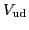values extracted by using diverse techniques including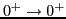nuclear decays, nuclear mirror decays, neutron decay, and pion decay are subject to both experimental and theoretical uncertainties. The latter pertain to calculations of radiative processes and - for nuclear methods - to the nuclear ISB effect. The uncertainties in radiative and ISB corrections affect the overall precision ofat the level of a few parts per 10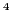each [1,52]. It should be stressed, however, that the ISB contribution to the error bar ofwas calculated only for a single theoretical model (SM-WS). Other microscopic models, including the SM-HF , RH-RPA , and projected DFT , yield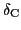corrections that may differ substantially from those obtained in SM-WS calculations. Inclusion of the model dependence in the calculated uncertainties is expected to increase the uncertainty ofby an order of magnitude ; of course, under the assumption that all the nuclear structure models considered are equally reliable.

A good way to verify the reliability of various models is to compare their predictions with empirically determined. Recently, an anomalously large value of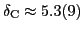% has been determined from a precision measurement of the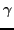yields following the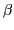-decay of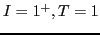state inCl to its isobaric analogue state (Fermi branch) inS . This value offers a stringent test on nuclear-structure models, because it is significantly larger than any value ofin the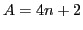nuclei. The physical reason for this enhancement can be traced back to a mixing of two close-lyingstates seen inS at the excitation energies of 7002keV and 7190keV, .

The experimental value% is consistent with the SM-WS calculations: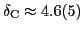%. In our projected-DFT approach, we also see fingerprints of the strong enhancement invalue inCl as compared to othernuclei. Unfortunately, a static DFT approach based on projecting from a single reference state is not sufficient to give a reliable prediction. This is because, as sketched in Fig. 14, there exist ambiguities in selecting the HF reference state. In the extreme isoscalar s.p. scenario, by distributing four valence protons and neutrons over the Nilsson s.p. levels in an odd-odd nucleus, one can form two distinctively different s.p. configurations, see Fig. 14.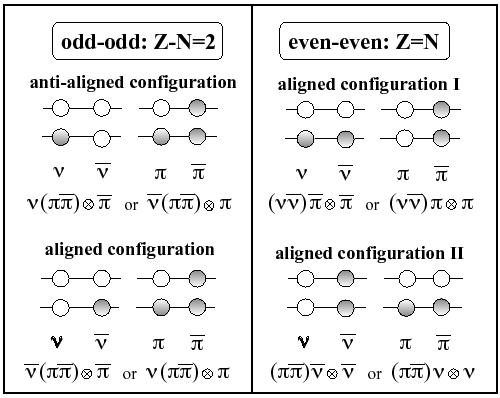The total signature of valence particles determines the total signature of the odd-odd nucleus and, in turn, an approximate angular-momentum distribution in its wave function ; the total additive signature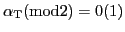corresponds then to even (odd) spins in the wave function . It is immediately seen that the anti-aligned configuration shown in Fig. 14 has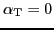; hence, in the first approximation, it can be disregarded. In this sense, the reference wave function inCl (or, in general, in any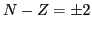odd-odd nucleus) corresponds to the uniquely defined aligned state. As seen in Fig. 14, this does not hold forS (or, in general, for any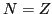even-even nucleus), where one must consider two possible Slater determinants having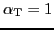, obtained by a suitable proton or neutron particle-hole excitation.

The above discussion indicates that, contrary to transitions involving the odd-oddnuclei studied in Sec. 3, those involving even-evennuclei cannot be directly treated within the present realization of the model. To this end, the model requires enhancements including the configuration mixing (multi-reference DFT). Nevertheless, we have carried out an exploratory study by independently calculating two ISB corrections for the two configurations discussed above. These calculations proceeded in the following way:

• We select the appropriate reference configurations which, in the present case, are: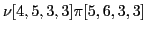inCl and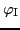: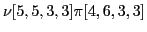and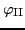: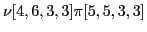inS. The labels denote the numbers of neutrons and protons occupying the lowest Nilsson levels in each parity-signature block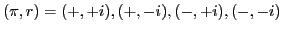counting from the bottom of the HF potential well, as defined in Ref. .
• We determine the lowest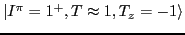and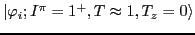(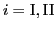) states by projecting onto subspaces of good angular momentum and isospin, and performing the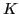-mixing and Coulomb rediagonalization as described in Sec. 2.
• Finally, we calculate matrix elements of the Fermi operator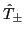and extract.

The resulting ISB corrections are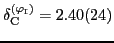% and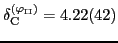% for the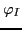andconfigurations, respectively. As before, we assumed a 10% error due to the basis size (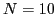spherical HO shells). Projections from the same configurations cranked in space to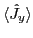= 1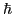(see discussion in Ref. ) leaves ISB corrections almost unaffected: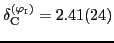% and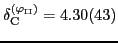%. A simple average value would read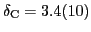%, which is indeed strongly enhanced as compared to the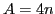cases, but still considerably smaller than both the empirical value and the SM-WS estimate. Whether or not the configuration-mixing calculations would provide a significant enhancement is an entirely open question.Next: Summary and perspectives Up: Isospin-breaking corrections to superallowed Previous: ISB corrections to the
Jacek Dobaczewski 2012-10-19